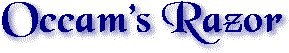At it's core, Occam's Razor assumes that simpler explanations are inherently "better" than complicated ones. The scientific method of hypothesis generation and testing relies heavily on this powerful tool. Here are some other interpretations:  One should always choose the simplest explanation of a phenomenon, the one that requires the fewest leaps of logic.  Don't make unnecessarily complicated assumptions.  Make things as simple as possible - but no simpler. (Albert Einstein) "KISS" - Keep It Simple, Stupid!  This logical principle is attributed to the mediaeval philosopher William of Occam. This principle, often also called the principle of parsimony, underlies all scientific modeling and theory building. It admonishes us to choose from a set of otherwise equivalent models of a given phenomenon the simplest one. In any given model, Occam's razor helps us to "cut away" those concepts, variables or constructs that are not really needed to explain the phenomenon. By doing that, developing the model will become much easier, and there is less chance of introducing inconsistencies, ambiguities and redundancies. Though the principle may seem rather trivial, it is essential for model building because of what is known as the "underdetermination of theories by data". For a given set of observations or data, there is always an infinite number of possible models explaining those same data. This is because a model normally represents an infinite number of possible cases, of which the observed cases are only a finite subset. The non-observed cases are inferred by postulating general rules covering both actual and potential observations.  For example, through two data points in a diagram you can always draw a straight line, and induce that all further observations will lie on that line. However, you could also draw an infinite variety of complex curves passing through those same two points, and those curves would fit the empirical data just as well. Occam's razor would, in this case, guide you to choose the "straight" (i.e. linear) relation as best candidate model. A similar reasoning can be made for n data points lying in any kind of distribution.  Occam's razor is especially important for universal models such as the ones developed in General Systems Theory, mathematics or philosophy, because there the subject domain is of an unlimited complexity. If one starts with too complicated foundations for a theory that potentially encompasses the universe, the chances of getting any manageable model are very slim indeed. Moreover, the principle is sometimes the only remaining guideline when entering domains of such a high level of abstraction that no concrete tests or observations can decide between rival models. In mathematical modeling of systems, the principle can be made more concrete in the form of the principle of uncertainty maximization: from your data, induce that model which minimizes the number of additional assumptions.  This principle is part of epistemology, and can be motivated by the requirement of maximal simplicity of cognitive models. However, its significance might be extended to metaphysics if it is interpreted as saying that simpler models are more likely to be correct than complex ones, in other words, that "nature" prefers simplicity.This page and the contents thereof are the property of Walker Mangum